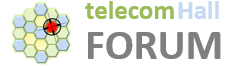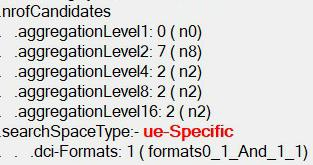# How to calculate minimum RB required in CORESET frequency resource (frequencyDomainResources)?

Gents,
How to calculate minimum RB required in CORESET frequency resource (frequencyDomainResources)?

It depends on aggregation level used and number of DCIs in one pdcch occasion. Ex. With AL4 and 2 DCIs, you will need 24*2=48 REGs or PRBs.

Thanks @Laxman.
Where can I know number of DCI in one occasion?

@Jaeku_Ryu gave calculation here, but only 1 sample.https://www.sharetechnote.com/html/5G/5G_ResourceAllocationUnit.html

It depends on the PDSCH scheduling.
How many PDSCH are we scheduling + some common DCI.

Number of DCIs are limited by gNB based on #AL and it’s size.
There can be few gNB parameters to calculate all that.

Can you help to calculate how many RBs required here?`(7*2+4*2+8*2+16*2)*6`

420 RB which is not possible as this is FR1.

That is max, but real depends on number of UEs scheduled in a slot.
In FR1 and 20 MHz you can have 106 * number of PDCCH symbols in a PDCCH occasion.

Why did you multiple by 6 at the end?

Ok let’s say with 100 MHz the max freq resource for CORESET should be only 273.

So do you mean we could not calculate it using above parameters?
How can we calculate then, can we check in MAC DCI?

Just for 273 resources: it is for one symbol.
Normally there are 2 or 3 symbols for PDCCH.
So 273*(2 or 3) for 100M.

Do You mean on UE side?
UE need not to know that.
gNB stop scheduling new UE when there are no more PDCCH resources available.

I mean for gNB side.
This has 1 symbol.
I’m talking about required RB for the CORESET.

So depends on AL used for each UE, once no resources remaining, no UE scheduled.
This is one of the parameters that limit #ue scheduled in a slot.

Can it be counted?

Yes, but depends on AL used for each UE (can be different).
Ex, 10M you have 48regs. You can have 2ues of AL4 or 8ues of AL2 or 1 ue of AL4 and 2UEs of AL2 , total 3UEs etc…

There are 45 bits Bitmap - if Bit is set equal to 6 then it indicates that 6 contiguous RB allocation within the downlink Bandwidth part.